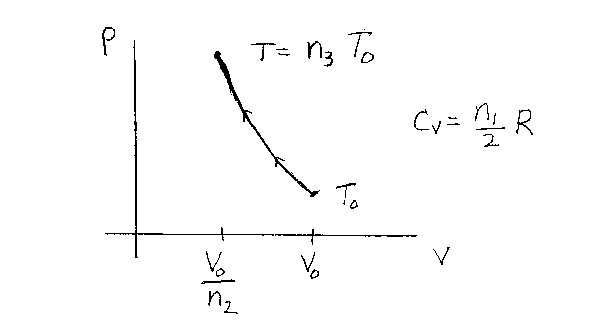Problem D17: An ideal gas starts out initially at a volume V0 and a temperature T0. It is then compressed adiabatically to a volume V0/n2. The molar heat capacity of the gas for a constant volume process is CV = n1R/2 for the temperatures in this problem. If the final temperature of the gas is T=n3T0, what is n3? Note that n1, n2 and n3 are unitless. Note that n1=3 for a monatomic gas, 5 for a diatomic gas, and 7 for a polyatomic gas in the temperature region of the problem. R is the gas constant.n1 = n2 = Input n3:

If you are currently in my class, you can record your grade by entering your name and student ID number (without the leading zeros) below and clicking on "record grade".
 First Name = Last Name = ID = Problem: